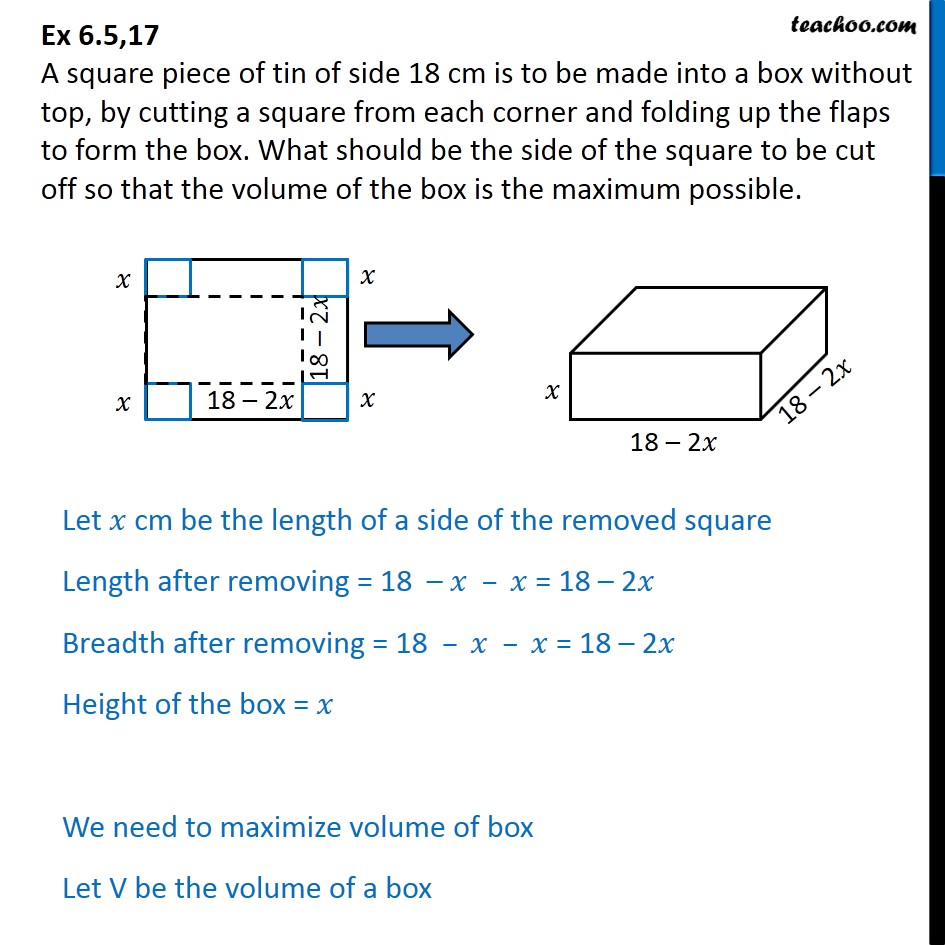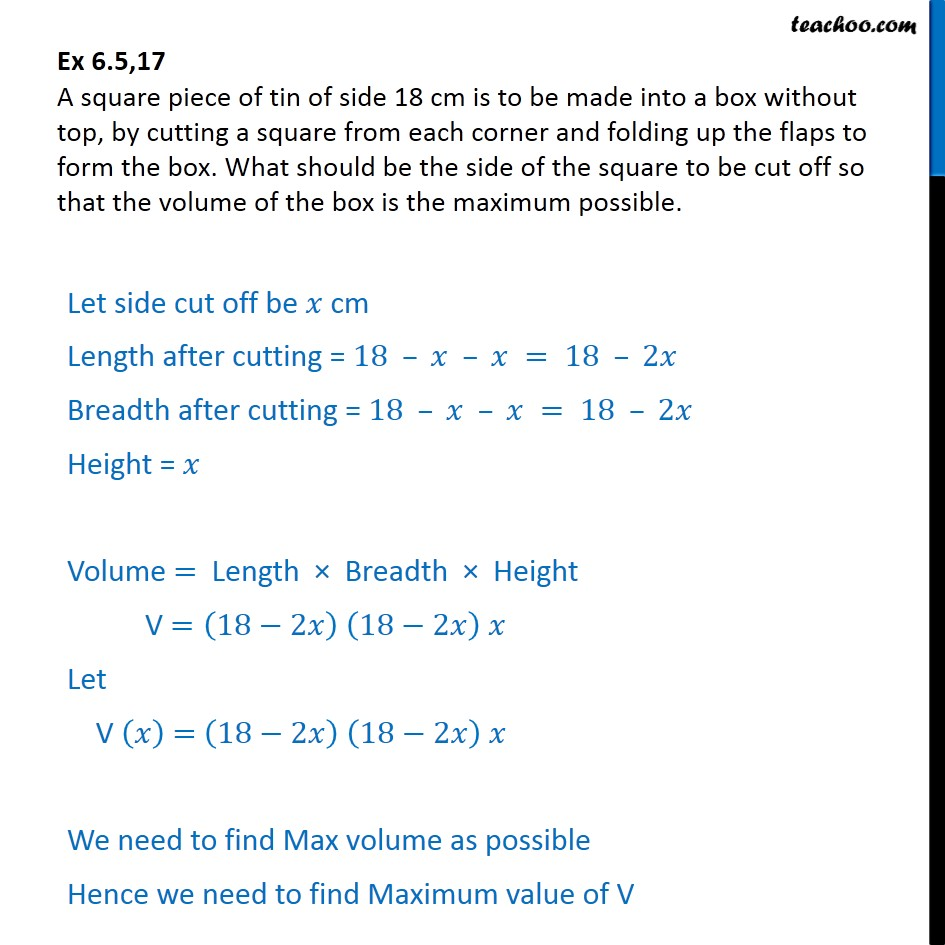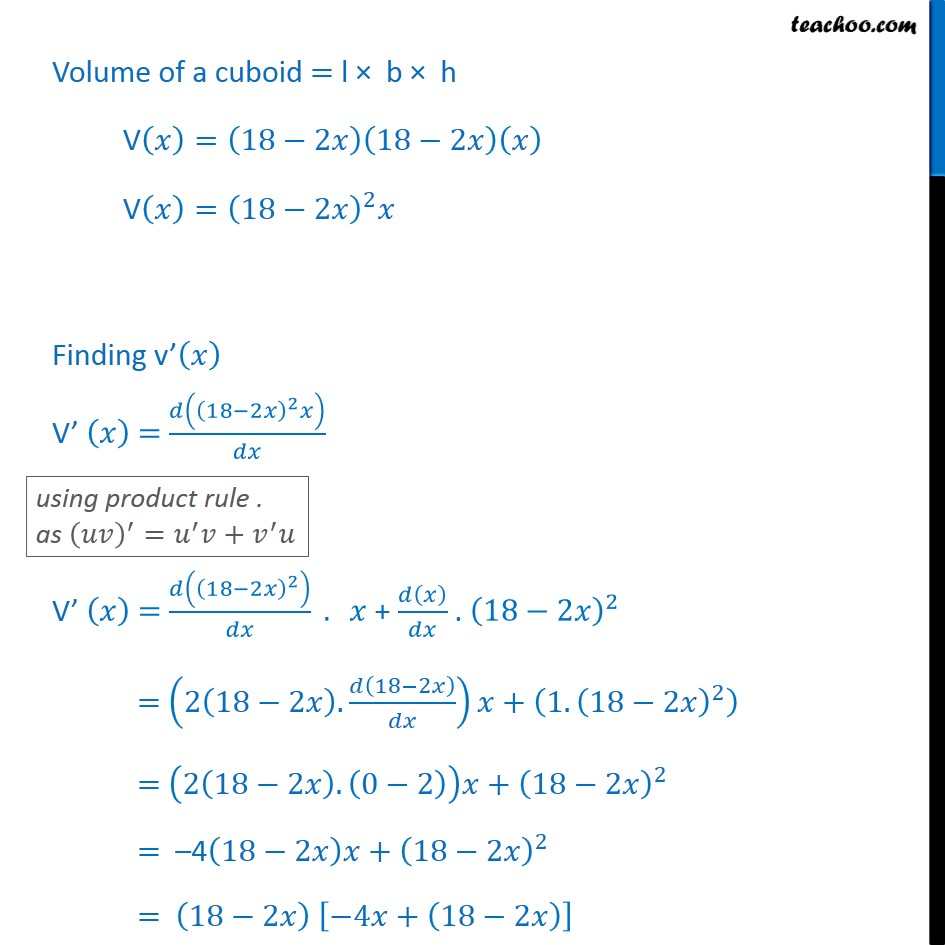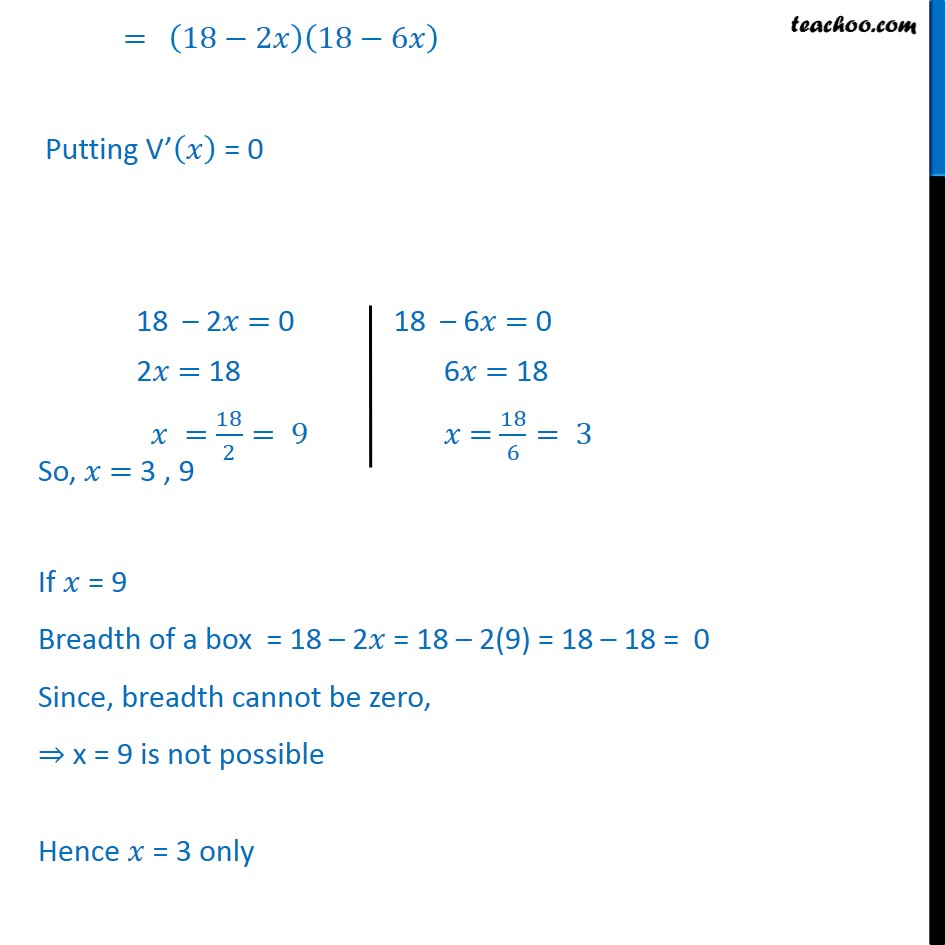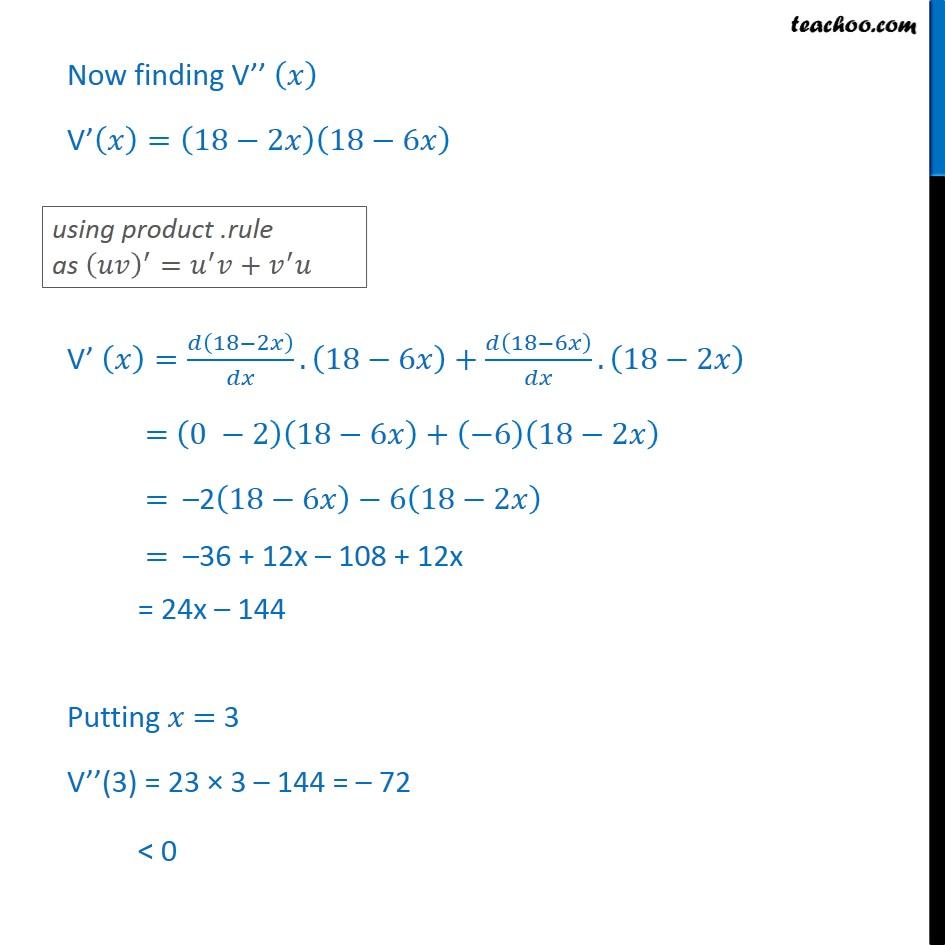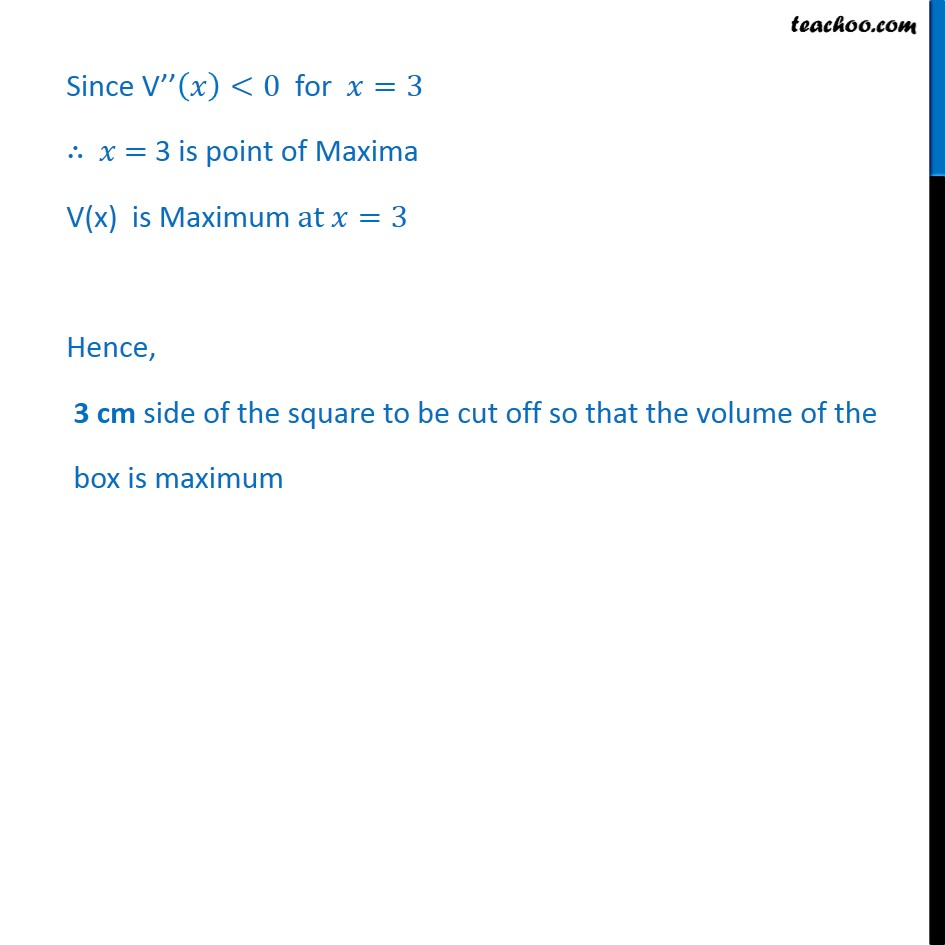1. Chapter 6 Class 12 Application of Derivatives
2. Serial order wise
3. Ex 6.5

Transcript

Ex 6.5,17 A square piece of tin of side 18 cm is to be made into a box without top, by cutting a square from each corner and folding up the flaps to form the box. What should be the side of the square to be cut off so that the volume of the box is the maximum possible. Let 𝑥 cm be the length of a side of the removed square Length after removing = 18 – 𝑥 – 𝑥 = 18 – 2𝑥 Breadth after removing = 18 – 𝑥 – 𝑥 = 18 – 2𝑥 Height of the box = 𝑥 We need to maximize volume of box Let V be the volume of a box Ex 6.5,17 A square piece of tin of side 18 cm is to be made into a box without top, by cutting a square from each corner and folding up the flaps to form the box. What should be the side of the square to be cut off so that the volume of the box is the maximum possible. Let side cut off be 𝑥 cm Length after cutting = 18 – 𝑥 – 𝑥 = 18 – 2𝑥 Breadth after cutting = 18 – 𝑥 – 𝑥 = 18 – 2𝑥 Height = 𝑥 Volume = Length × Breadth × Height V =﷐18−2𝑥﷯ ﷐18−2𝑥﷯ 𝑥 Let V ﷐𝑥﷯ = ﷐18−2𝑥﷯ ﷐18−2𝑥﷯ 𝑥 We need to find Max volume as possible Hence we need to find Maximum value of V Volume of a cuboid = l × b × h V﷐𝑥﷯=﷐18−2𝑥﷯﷐18−2𝑥﷯﷐𝑥﷯ V﷐𝑥﷯=﷐﷐18−2𝑥﷯﷮2﷯𝑥 Finding v’﷐𝑥﷯ V’ ﷐𝑥﷯ = ﷐𝑑﷐﷐﷐18−2𝑥﷯﷮2﷯𝑥﷯﷮𝑑𝑥﷯ V’ ﷐𝑥﷯ = ﷐𝑑﷐﷐﷐18−2𝑥﷯﷮2﷯﷯﷮𝑑𝑥﷯ . 𝑥 + ﷐𝑑﷐𝑥﷯﷮𝑑𝑥﷯ . ﷐﷐18−2𝑥﷯﷮2﷯ = ﷐2﷐18−2𝑥﷯.﷐𝑑﷐18−2𝑥﷯﷮𝑑𝑥﷯﷯𝑥+﷐1.﷐﷐18−2𝑥﷯﷮2﷯﷯ = ﷐2﷐18−2𝑥﷯.﷐0−2﷯﷯𝑥+ ﷐﷐18−2𝑥﷯﷮2﷯ = –4﷐18−2𝑥﷯𝑥+﷐﷐18−2𝑥﷯﷮2﷯ = ﷐18−2𝑥﷯ ﷐−4𝑥+﷐18−2𝑥﷯﷯ = ﷐18−2𝑥﷯﷐18−6𝑥﷯ Putting V’﷐𝑥﷯ = 0 So, 𝑥 = 3 , 9 If 𝑥 = 9 Breadth of a box = 18 – 2𝑥 = 18 – 2(9) = 18 – 18 = 0 Since, breadth cannot be zero, ⇒ x = 9 is not possible Hence 𝑥 = 3 only Now finding V’’ ﷐𝑥﷯ V’﷐𝑥﷯=﷐18−2𝑥﷯﷐18−6𝑥﷯ V’ ﷐𝑥﷯=﷐𝑑﷐18−2𝑥﷯﷮𝑑𝑥﷯.﷐18−6𝑥﷯+﷐𝑑﷐18−6𝑥﷯﷮𝑑𝑥﷯.﷐18−2𝑥﷯ = ﷐0 −2﷯﷐18−6𝑥﷯+﷐−6﷯﷐18−2𝑥﷯ = –2﷐18−6𝑥﷯−6﷐18−2𝑥﷯ = –36 + 12x – 108 + 12x = 24x – 144 Putting 𝑥 = 3 V’’(3) = 23 × 3 – 144 = – 72 < 0 Since V’’﷐𝑥﷯<0 for 𝑥=3 ∴ 𝑥 = 3 is point of Maxima V(x) is Maximum at 𝑥=3 Hence, 3 cm side of the square to be cut off so that the volume of the box is maximum

Ex 6.5